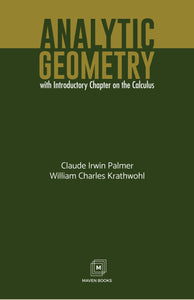# ANALYTIC GEOMETRY With Introductory Chapter on the Calculus

Vendor

MJP PUBLISHERS

Type

Books

Categories
140 in stock.
Quantity must be 1 or more
Regular price Rs. 595.00

Introduction, Geometric facts expressed Analytically, and Conversely, Loci and Equations, The straight line and the general equation of the first degree, The circle and certain forms of the second degree equation, The Parabola and certain forms of the second degree equation, The Ellipse and certain forms of the second degree equation, The Hyperbola and certain forms of the second degree equation, Other Loci and equations, Empirical Loci and equations, Poles, Polars and diameters, Elements of calculus, Solid Analytic Geometry,
Sale

Unavailable

Sold Out